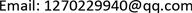﻿ 一致模糊偏序集及其应用 Uniformly Fuzzy Posets and Its Applications

Pure Mathematics
Vol. 08  No. 06 ( 2018 ), Article ID: 27602 , 5 pages
10.12677/PM.2018.86091

Uniformly Fuzzy Posets and Its Applications

Hui Li, Lu Chen

School of Mathematical Sciences, Huaibei Normal University, Huaibei AnhuiReceived: Oct. 25th, 2018; accepted: Nov. 6th, 2018; published: Nov. 16th, 2018ABSTRACT

In this paper, the concept of uniform fuzzy posets is introduced on the fuzzy directed posets, and the basic properties of the uniformly fuzzy posets are discussed. Secondly, the uniformly fuzzy complete posets are introduced on the basis of uniformly fuzzy posets. Finally, by introducing a new fuzzy way-below relation, the concept of uniformly fuzzy continuous posets is introduced and some properties are given.

Keywords:Uniformly Fuzzy Posets, Uniformly Fuzzy Complete Posets, Uniformly Fuzzy Continuous Posets1. 引言

1965年Zadeh, L.A.提出模糊集  的概念，它的提出标志着模糊数学的诞生。模糊数学是传统数学的一个推广。文献  讨论了模糊偏序集上的若干性质，得到了若干好的等价刻画。文献  提出模糊DCPO的概念，给出了若干好的性质，然后在模糊DCPO的基础上引入模糊Domain的概念，进而得到了模糊Domain上的若干重要结果。文献  给出了模糊半连续格的概念，并讨论了其上的一些范畴性质。文献  在模糊Dcpo的基础上讨论了模糊Domain上的Ω范畴性质。文献  引入完备模糊格的概念，给出模糊完备格上若干好的性质。本文在以上文献基础上引入一致模糊集和一致模糊完备集的概念，讨论其上的若干性质。其次在一致模糊完备的基础上利用新的模糊way-below关系给出一致模糊Domain的概念，探讨其若干性质。一致模糊集是模糊定向集的一个推广。

2. 预备

i) 自反性： $\forall x\in X$$e\left(x,x\right)=1$

ii) 传递性： $\forall x,y,z\in X$$e\left(x,y\right)\wedge e\left(y,z\right)\le e\left(x,z\right)$

iii) 反对称性： $\forall x,y\in X$$e\left(x,y\right)=e\left(y,x\right)=1⇒x=y$

$su{b}_{X}\left(A,B\right)=\underset{x\in X}{\wedge }A\left(x\right)\to B\left(x\right)$

1) $\forall x\in X$$A\left(x\right)\le e\left(x,{x}_{0}\right)$ ；(相应的， $\forall x\in X$$A\left(x\right)\le e\left({x}_{0},x\right)$ )

2) $\forall y\in X$$\underset{x\in X}{\wedge }\left(A\left(x\right)\to e\left(x,y\right)\right)\le e\left({x}_{0},y\right)$

(相应的， $\forall y\in X$$\underset{x\in X}{\wedge }\left(A\left(x\right)\to e\left(y,x\right)\right)\le e\left(y,{x}_{0}\right)$ )，则称 ${x}_{0}$ 为A的上确界(相应的，下确界)，记作 ${x}_{0}=\coprod A$ (相应的， ${x}_{0}=\prod A$ )。

$\forall y\in X$$↓A\left(x\right)=\underset{y\in X}{\vee }A\left(y\right)\wedge e\left(x,y\right)$ ( $↑A\left(x\right)=\underset{y\in X}{\vee }A\left(y\right)\wedge e\left(y,x\right)$ ).

1) $\underset{x\in X}{\vee }D\left(x\right)=1$ ；2) $\forall x,y\in X$$D\left(x\right)\wedge D\left(y\right)\le \underset{z\in X}{\vee }D\left(z\right)\wedge e\left(x,z\right)\wedge e\left(y,z\right)$

3. 主要结果

1) $\underset{x\in X}{\vee }A\left(x\right)=1$

2) $D\left(x\right)\wedge D\left(y\right)\le \underset{z\in X}{\vee }A\left(z\right)\wedge e\left(x,z\right)\wedge e\left(y,z\right)$

${⇓}_{UF}x\left(y\right)=\underset{I\in UFI\left(X\right)}{\wedge }\left(e\left(x,\coprod I\right)\to I\left(y\right)\right)$ .

${⇓}_{UF}:X×X\to L$$\left(X,e\right)$ 上的一致模糊way-below关系，且 ${⇓}_{UF}x\left(y\right)=\text{\hspace{0.17em}}{⇓}_{UF}\left(y,x\right)$ 。如果 $\forall x\in X$${⇓}_{UF}x\in UF\left(X\right)$$x=\text{\hspace{0.17em}}{⇓}_{UF}\coprod x$ ，则称 $\left(X,e\right)$ 为一致模糊连续偏序集或一致模糊Domain。

${⇓}_{UF}x\left(y\right)\le e\left(x,\coprod A\right)\to \text{\hspace{0.17em}}↓A\left(y\right)=1\to ↓A\left(y\right)=\text{\hspace{0.17em}}↓A\left(y\right)$ .

1) $\underset{y\in X}{\wedge }e\left(x,y\right)\le I\left(x\right)$

2) $\underset{z\in X}{\wedge }e\left(x,y\right)\le \text{\hspace{0.17em}}{⇓}_{UF}y\left(x\right)$

3) ${⇓}_{UF}x\le \text{\hspace{0.17em}}↓x$

4) 对任意的 $u,v\in X,e\left(u,x\right)\wedge {⇓}_{UF}y\left(x\right)\wedge e\left(y,v\right)\le \text{\hspace{0.17em}}{⇓}_{UF}v\left(u\right)$

1) $\underset{y\in X}{\wedge }e\left(x,y\right)=\left(\underset{y\in X}{\wedge }e\left(x,y\right)\right)\wedge \left(\underset{z\in X}{\vee }I\left(z\right)\right)=\underset{z\in X}{\vee }\left(\underset{y\in X}{\wedge }e\left(x,y\right)\wedge I\left(z\right)\right)\le \underset{z\in X}{\vee }\left(e\left(x,z\right)\wedge I\left(z\right)\right)\le I\left(x\right)$

2) ${⇓}_{UF}y\left(x\right)=\underset{I\in UFI\left(X\right)}{\wedge }e\left(y,\coprod I\right)\to I\left(x\right)\ge \underset{I\in UFI\left(X\right)}{\wedge }I\left(x\right)\ge \underset{z\in X}{\wedge }e\left(x,z\right)$

3) ${⇓}_{UF}x\left(y\right)\le e\left(x,\coprod ↓x\right)\to \text{\hspace{0.17em}}↓x\left(y\right)=e\left(x,x\right)\to e\left(y,x\right)=e\left(y,x\right)=\text{\hspace{0.17em}}↓x\left(y\right)$

4) $\begin{array}{l}e\left(v,\coprod I\right)\wedge e\left(u,x\right)\wedge e\left(y,u\right)\wedge \left(e\left(y,\coprod I\right)\to I\left(x\right)\right)\le e\left(y,\coprod I\right)\wedge \left(e\left(y,\coprod I\right)\to I\left(x\right)\right)\wedge e\left(u,x\right)\\ \text{\hspace{0.17em}}\text{\hspace{0.17em}}\text{\hspace{0.17em}}\text{\hspace{0.17em}}\text{\hspace{0.17em}}\text{\hspace{0.17em}}\text{\hspace{0.17em}}\text{\hspace{0.17em}}\text{\hspace{0.17em}}\text{\hspace{0.17em}}\text{\hspace{0.17em}}\text{\hspace{0.17em}}\text{\hspace{0.17em}}\text{\hspace{0.17em}}\text{\hspace{0.17em}}\text{\hspace{0.17em}}\text{\hspace{0.17em}}\text{\hspace{0.17em}}\text{\hspace{0.17em}}\text{\hspace{0.17em}}\text{\hspace{0.17em}}\text{\hspace{0.17em}}\text{\hspace{0.17em}}\text{\hspace{0.17em}}\text{\hspace{0.17em}}\text{\hspace{0.17em}}\text{\hspace{0.17em}}\text{\hspace{0.17em}}\text{\hspace{0.17em}}\text{\hspace{0.17em}}\text{\hspace{0.17em}}\text{\hspace{0.17em}}\text{\hspace{0.17em}}\text{\hspace{0.17em}}\text{\hspace{0.17em}}\text{\hspace{0.17em}}\text{\hspace{0.17em}}\text{\hspace{0.17em}}\text{\hspace{0.17em}}\text{\hspace{0.17em}}\text{\hspace{0.17em}}\text{\hspace{0.17em}}\text{\hspace{0.17em}}\text{\hspace{0.17em}}\text{\hspace{0.17em}}\text{\hspace{0.17em}}\text{\hspace{0.17em}}\text{\hspace{0.17em}}\text{\hspace{0.17em}}\text{\hspace{0.17em}}\text{\hspace{0.17em}}\text{\hspace{0.17em}}\text{\hspace{0.17em}}\text{\hspace{0.17em}}\text{\hspace{0.17em}}\text{\hspace{0.17em}}\text{\hspace{0.17em}}\text{\hspace{0.17em}}\text{\hspace{0.17em}}\text{\hspace{0.17em}}\text{\hspace{0.17em}}\text{\hspace{0.17em}}\text{\hspace{0.17em}}\text{\hspace{0.17em}}\text{\hspace{0.17em}}\text{\hspace{0.17em}}\le e\left(u,x\right)\wedge I\left(x\right)\le I\left(u\right)\end{array}$

$e\left(u,x\right)\wedge e\left(y,u\right)\wedge \left(e\left(y,\coprod I\right)\to I\left(x\right)\right)\le e\left(v,\coprod I\right)\to I\left(u\right)$

${⇓}_{UF}y\left(x\right)\le \underset{z\in X}{\vee }{⇓}_{UF}z\left(x\right)\wedge {⇓}_{UF}y\left(z\right)$ .

$\forall a\in X$ ，令 $A\left(a\right)=\underset{z\in X}{\vee }{⇓}_{UF}z\left(x\right)\wedge {⇓}_{UF}y\left(z\right)$ 。故只需证 ${⇓}_{UF}y\left(a\right)\le A\left(x\right)$

1) $\begin{array}{c}\underset{a\in X}{\vee }A\left(a\right)=\underset{a\in X}{\vee }\underset{z\in X}{\vee }{⇓}_{UF}z\left(a\right)\wedge {⇓}_{UF}y\left(z\right)=\underset{z\in X}{\vee }\underset{a\in X}{\vee }{⇓}_{UF}z\left(a\right)\wedge {⇓}_{UF}y\left(z\right)\\ =\underset{z\in X}{\vee }\left({⇓}_{UF}y\left(z\right)\wedge \left(\underset{a\in X}{\vee }{⇓}_{UF}z\left(a\right)\right)\right)=\underset{z\in X}{\vee }{⇓}_{UF}y\left(z\right)=1\end{array}$

2) $\forall a,b\in X$

$A\left(a\right)\wedge e\left(b,a\right)=\underset{z\in X}{\vee }{⇓}_{UF}z\left(a\right)\wedge {⇓}_{UF}y\left(z\right)\wedge e\left(b,a\right)\le \underset{z\in X}{\vee }{⇓}_{UF}z\left(b\right)\wedge {⇓}_{UF}y\left(z\right)=A\left(b\right)$ .

3) $\forall a,b\in X$$\forall D\subseteq A$ ，由命题3.1 (4)，

$\begin{array}{l}D\left(a\right)\wedge D\left(b\right)\\ =\underset{{a}_{1},{b}_{1}\in X}{\vee }{⇓}_{UF}{a}_{1}\left(a\right)\wedge {⇓}_{UF}{b}_{1}\left(b\right)\wedge {⇓}_{UF}y\left({a}_{1}\right)\wedge {⇓}_{UF}y\left({b}_{1}\right)\\ \le \underset{{a}_{1},{b}_{1}\in X}{\vee }\underset{c\in X}{\vee }{⇓}_{UF}{a}_{1}\left(a\right)\wedge {⇓}_{UF}{b}_{1}\left(b\right)\wedge {⇓}_{UF}y\left(c\right)\wedge e\left(a,c\right)\wedge e\left(b,c\right)\\ =\underset{{a}_{1},{b}_{1}\in X}{\vee }\underset{c\in X}{\vee }\left({⇓}_{UF}{a}_{1}\left(a\right)\wedge e\left(a,c\right)\right)\wedge \left({⇓}_{UF}{b}_{1}\left(b\right)\wedge e\left(b,c\right)\right)\wedge {⇓}_{UF}y\left(c\right)\\ \le \underset{{a}_{1},{b}_{1}\in X}{\vee }\underset{c\in X}{\vee }{⇓}_{UF}c\left(a\right)\wedge {⇓}_{UF}c\left(b\right)\wedge {⇓}_{UF}y\left(c\right)\end{array}$

$\begin{array}{l}=\underset{c\in X}{\vee }{⇓}_{UF}c\left(a\right)\wedge {⇓}_{UF}c\left(b\right)\wedge {⇓}_{UF}y\left(c\right)\\ \le \underset{c\in X}{\vee }\underset{d\in X}{\vee }{⇓}_{UF}c\left(d\right)\wedge e\left(a,d\right)\wedge e\left(b,d\right)\wedge {⇓}_{UF}y\left(c\right)\\ =\underset{d\in X}{\vee }\left(e\left(a,d\right)\wedge e\left(b,d\right)\wedge \left(\underset{c\in X}{\vee }{⇓}_{UF}c\left(d\right)\wedge {⇓}_{UF}y\left(c\right)\right)\right)\\ =\underset{d\in X}{\vee }e\left(a,d\right)\wedge e\left(b,d\right)\wedge A\left(d\right)\end{array}$

$\begin{array}{l}\underset{z\in X}{\wedge }A\left(z\right)\to e\left(z,a\right)=\underset{z\in X}{\wedge }\underset{{a}_{1}\in X}{\wedge }\left({⇓}_{UF}{a}_{1}\left(z\right)\wedge {⇓}_{UF}y\left({a}_{1}\right)\to e\left(z,a\right)\right)\\ \text{\hspace{0.17em}}\text{\hspace{0.17em}}\text{\hspace{0.17em}}\text{\hspace{0.17em}}\text{\hspace{0.17em}}\text{\hspace{0.17em}}\text{\hspace{0.17em}}\text{\hspace{0.17em}}\text{\hspace{0.17em}}\text{\hspace{0.17em}}\text{\hspace{0.17em}}\text{\hspace{0.17em}}\text{\hspace{0.17em}}\text{\hspace{0.17em}}\text{\hspace{0.17em}}\text{\hspace{0.17em}}\text{\hspace{0.17em}}\text{\hspace{0.17em}}\text{\hspace{0.17em}}\text{\hspace{0.17em}}\text{\hspace{0.17em}}\text{\hspace{0.17em}}\text{\hspace{0.17em}}\text{\hspace{0.17em}}\text{\hspace{0.17em}}\text{ }\text{ }=\underset{{a}_{1}\in X}{\wedge }\left({⇓}_{UF}y\left({a}_{1}\right)\to \underset{z\in X}{\wedge }\left({⇓}_{UF}{a}_{1}\left(z\right)\to e\left(z,a\right)\right)\right)\\ \text{\hspace{0.17em}}\text{\hspace{0.17em}}\text{\hspace{0.17em}}\text{\hspace{0.17em}}\text{\hspace{0.17em}}\text{\hspace{0.17em}}\text{\hspace{0.17em}}\text{\hspace{0.17em}}\text{\hspace{0.17em}}\text{\hspace{0.17em}}\text{\hspace{0.17em}}\text{\hspace{0.17em}}\text{\hspace{0.17em}}\text{\hspace{0.17em}}\text{\hspace{0.17em}}\text{\hspace{0.17em}}\text{\hspace{0.17em}}\text{\hspace{0.17em}}\text{\hspace{0.17em}}\text{\hspace{0.17em}}\text{\hspace{0.17em}}\text{\hspace{0.17em}}\text{\hspace{0.17em}}\text{\hspace{0.17em}}\text{\hspace{0.17em}}\text{ }\text{ }=\underset{{a}_{1}\in X}{\wedge }{⇓}_{UF}y\left({a}_{1}\right)\to e\left(\coprod {⇓}_{UF}{a}_{1},a\right)\\ \text{\hspace{0.17em}}\text{\hspace{0.17em}}\text{\hspace{0.17em}}\text{\hspace{0.17em}}\text{\hspace{0.17em}}\text{\hspace{0.17em}}\text{\hspace{0.17em}}\text{\hspace{0.17em}}\text{\hspace{0.17em}}\text{\hspace{0.17em}}\text{\hspace{0.17em}}\text{\hspace{0.17em}}\text{\hspace{0.17em}}\text{\hspace{0.17em}}\text{\hspace{0.17em}}\text{\hspace{0.17em}}\text{\hspace{0.17em}}\text{\hspace{0.17em}}\text{\hspace{0.17em}}\text{\hspace{0.17em}}\text{\hspace{0.17em}}\text{\hspace{0.17em}}\text{\hspace{0.17em}}\text{\hspace{0.17em}}\text{\hspace{0.17em}}\text{ }\text{ }=\underset{{a}_{1}\in X}{\wedge }{⇓}_{UF}y\left({a}_{1}\right)\to e\left({a}_{1},a\right)=e\left(y,a\right)\end{array}$ .

1) 必要性。 $\forall x,y\in X$

$\begin{array}{c}sub\left({⇓}_{UF}x,{⇓}_{UF}y\right)=\underset{z\in X}{\wedge }\left(\underset{I\in UFI\left(X\right)}{\wedge }e\left(x,\coprod I\right)\to I\left(z\right)\right)\to \left(\underset{J\in UFI\left(X\right)}{\wedge }e\left(y,\coprod J\right)\to J\left(z\right)\right)\\ \ge \underset{z\in X}{\wedge }\left(\underset{J\in UFI\left(X\right)}{\wedge }e\left(y,\coprod I\right)\to J\left(z\right)\right)\to \left(e\left(y,\coprod J\right)\to J\left(z\right)\right)\\ \ge \underset{J\in UFI\left(X\right)}{\wedge }e\left(y,\coprod J\right)\to e\left(x,\coprod J\right)\ge e\left(x,y\right)\end{array}$ .

${⇓}_{UF}$ 是保模糊序的。 $\forall I,J\in UFI\left(X\right)$

$e\left(\coprod I,\coprod J\right)=\underset{x\in X}{\wedge }I\left(x\right)\to e\left(x,\coprod J\right)\ge \underset{x\in X}{\wedge }I\left(x\right)\to J\left(x\right)=su{b}_{X}\left(I,J\right)$ ，故 $\coprod$ 也是保模糊序的。

$\forall x\in X,I\in UFI\left(X\right)$ ,

$su{b}_{X}\left({⇓}_{UF}x,I\right)=\underset{y\in X}{\wedge }{⇓}_{UF}x\left(y\right)\to I\left(y\right)\ge \underset{y\in I}{\wedge }\left(e\left(x,\coprod I\right)\to I\left(y\right)\right)\to I\left(y\right)\ge e\left(x,\coprod I\right)$ .

2) 充分性。因 $\left({⇓}_{UF},\coprod \right)$$\left(X,e\right)$$\left(UFI\left(X\right),su{b}_{X}\right)$ 之间的一个模糊Galois伴随，故 $\forall x\in X$${⇓}_{UF}x\in UFI\left(X\right)$$e\left(x,\coprod {⇓}_{UF}x\right)=1$ ，进而 $x\le \coprod {⇓}_{UF}x\le \coprod ↓x=x$ ，故 $x=\coprod {⇓}_{UF}x$ 。所以 $\left(X,e\right)$ 是一致模糊Domain。

Uniformly Fuzzy Posets and Its Applications[J]. 理论数学, 2018, 08(06): 676-680. https://doi.org/10.12677/PM.2018.86091

1. 1. Zadeh, L.A. (1965) Fuzzy Sets. Information and Control, 8, 338-353.
https://doi.org/10.1016/S0019-9958(65)90241-X

2. 2. 张奇业. L-Fuzzy Domain理论[D]: [博士学位论文]. 北京: 首都师范大学, 2002.

3. 3. Yao, W. and Shi, F.G. (2010) Quantitative Domain via Fuzzy Sets: Part I: Continuity of Fuzzy Directed Complete Posets. Fuzzy Sets and Systems, 161, 973-987.
https://doi.org/10.1016/j.fss.2009.06.018

4. 4. 韩艳伟. 模糊半连续格及其范畴性质[D]: [硕士学位论文]. 西安: 陕西师范大学, 2011.

5. 5. Lai, H. and Zhang, D. (2007) Complete and Directed Complete Ω-Categories. Theoretical Computer Science, 388, 1-25.
https://doi.org/10.1016/j.tcs.2007.09.012

6. 6. Lai, H.L. (2005) Complete Fuzzy Lattice. Foundations of Fuzzy Set Theory, Beijing, IFSA: 246-251.

7. 7. Yao, W. and Lu, L.X. (2009) Fuzzy Galois Connections on Fuzzy Posets. Mathematical Logic Quarterly, 55, 84-91.
https://doi.org/10.1002/malq.200710079